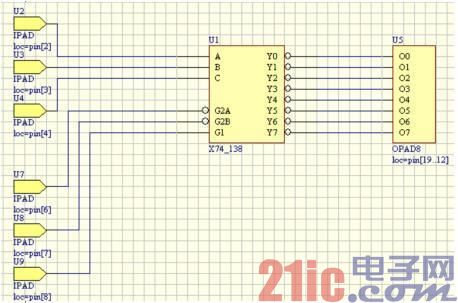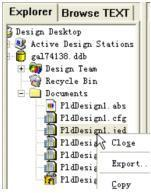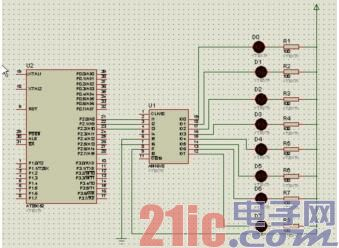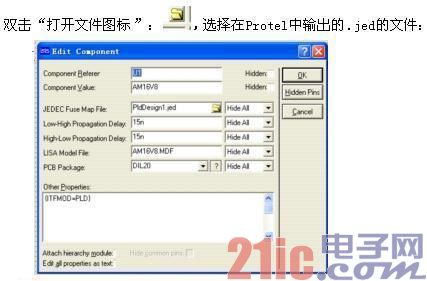### PLD的PROTEUS电路仿真

1PROTEUS软件简介

PROTEUS是来自英国公司的工具软件，在全球广泛使用。和其它工具相比，这款软件的最大特点就在于它能够模拟单片机。可以直接在基于原理图的虚拟原型EDA上编程，并实现软件源码级的实时调试。还能看到运行后输入输出的效果。但是现在大家都只注意到PROTEUS对单片机的仿真和如何与Keil进行关联调试，其实PROTEUS还能够进行PLD的电路仿真。

PLD(可编程逻辑器件)是一种数字集成电路的半成品，在其芯片上按一定排列方式集成了大量的门和触发器等基本逻辑元件，使用者可利用某种开发工具对其进行加工，即按设计要求将这些片内的元件连接起来，使之完成某个逻辑电路或系统的功能，成为一个可在实际电子系统中使用的专用集成电路。

2. PLD的设计Protel 99SE的PLD原理图的设计与普通原理图相同，但有几点是需要注意：3 Proteus对PLD的仿真4.结语

#include sbit P2_0=P2^0；sbit P2_1=P2^1；sbit P2_2=P2^2；

void delaytime（int count）；void main（void）

{ while（1）

{ P2_2=0；P2_1=0；P2_0=0；delaytime（500）；P2_2=0；P2_1=0；P2_0=1；delaytime（500）；P2_2=0；P2_1=1；P2_0=0；delaytime（500）；P2_2=0；P2_1=1；P2_0=1；delaytime（500）；P2_2=1；P2_1=0；P2_0=0；delaytime（500）；P2_2=1；P2_1=0；P2_0=1；delaytime（500）；P2_2=1；P2_1=1；P2_0=0；delaytime（500）；P2_2=1；P2_1=1；P2_0=1；delaytime（500）；

}

void delaytime(int count)

{

int j,k;

while(count-- !=0)

{

for(j=0;j<10;j++)

for(k=0;k<72;k++)

;

}

}

## 课程推荐» 更多

x